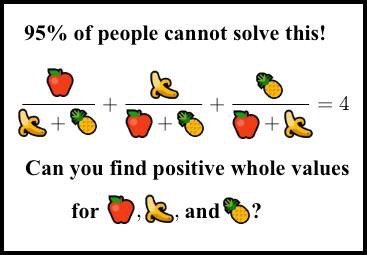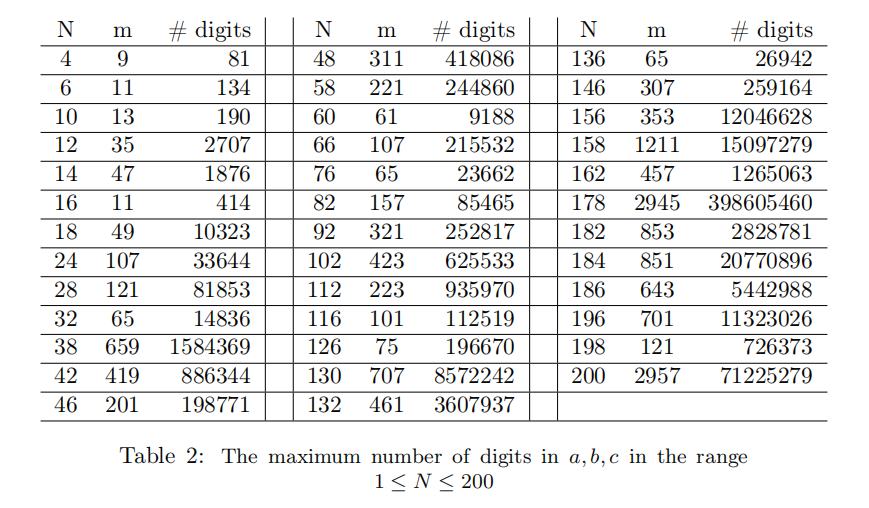# 求解 a/(b+c) + b/(a+c) + c/(a+b) = 4 的正整数解a = 154476802108746166441951315019919837485664325669565431700026634898253202035277999
b = 36875131794129999827197811565225474825492979968971970996283137471637224634055579
c = 4373612677928697257861252602371390152816537558161613618621437993378423467772036

• $N$ 为奇数时，方程没有正整数解（但可能有整数解）。这个也许有更简便的证明方法。
• $N$ 为偶数时，方程可能有也可能没有正整数解，但有解的时候，解也可能超级大。有多超级呢，当$N=896$时，最小的解都有几万亿位（注意是几万亿位，而不是几万亿）。Q. E. D.

##### 类似文章：

IBM Ponder this 每个月会出一道谜题。这个月的题目是求所有的整数，它既是一个平方数，又是一个 triangular number （即可以表示为$1+2+\cdots+m$）。